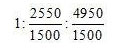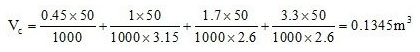# Calculate Quantities of Materials for ConcreteAbsolute volume method is considered as the most precise method for estimating the amount of materials which are used for producing essential amount of concrete for disposed mix proportions.

This method is formed by following the principle that the volume of entirely condensed concrete is equivalent to the absolute volume of all the materials required for concrete formation which range from cement, sand, coarse aggregates and water.

Given below the formula for computing the materials mandatory for volume of concrete:

Where, Vc = Absolute volume of fully compacted fresh concrete
W =Mass of water
C = Mass of cement
Fa = Mass of fine aggregates
Ca = Mass of coarse aggregates
Sc, Sfa and Sca refer to the definite gravities of cement, fine aggregates and coarse aggregates correspondingly.

The air content is not included in this estimation.

This estimation process toward quantities of materials for concrete involves the mix proportions from design mix or nominal mixes for structural stability and longevity requirement.

Now the focus will be given on the material calculation through an example.

Select concrete containing mix proportion of 1:1.5:3 where, 1 denotes part of cement, 1.5 denotes part of fine aggregates and 3 denotes part of coarse aggregates of maximum size of 20mm. The water cement ratio necessary for concrete alloy is assumed as 0.45.

Bulk densities of materials are considered as follows:

Cement = 1500 kg/m3
Sand = 1700 kg/m3
Coarse aggregates = 1650 kg/m3

Specific gravities of concrete materials are as follows:

Cement = 3.15
Sand = 2.6
Coarse aggregates = 2.6.
The percentage of entrained air is supposed to 2%.
The mix proportion of 1:1.5:3 through dry volume of materials are defined in terms of masses as:
Cement = 1 x 1500 = 1500
Sand = 1.5 x 1700 = 2550
Coarse aggregate = 3 x 1650 = 4950.
Consequently, the ratio of masses of these materials w.r.t. cement will be defined like == 1 : 1.7 : 3.3
The water cement ratio = 0.45

In order to calculate the volume of concrete that is based on one bag of cement (i.e. 50 kg cement) in support of the mass proportions of concrete materials.

Thus, the absolute volume of concrete for 50 kg of cement =As a result, for the proportion of blend measured, with 3 bag of cement of 50 kg, 0.1345 m3 of concrete will be generated.

Here an entrained air of 2% is included. Therefore the substantial volume of concrete for 1 cubic meter of consolidated concrete construction is calculated to be = 1 -0.02 = 0.98 m3.

Therefore, the required amount of cement for 1 cubic meter of concrete is calculated to be = 0.98/0.1345 = 7.29 bags of cement.

The following calculation is required to find out the quantities of materials for 1 m3 of concrete production:

The weight of cement required = 7.29 x 50 = 364.5 kg.
Weight of fine aggregate (sand) = 1.5 x 364.5 = 546.75 kg.
Weight of coarse aggregate = 3 x 364.5 = 1093.5 kg.